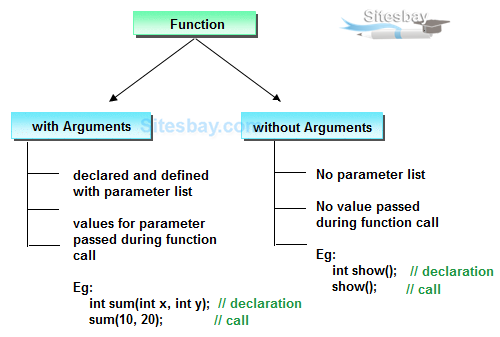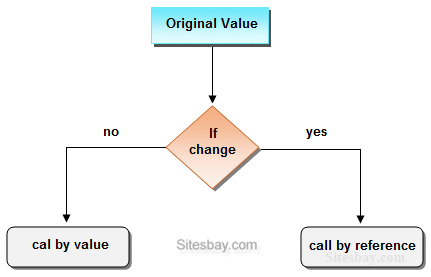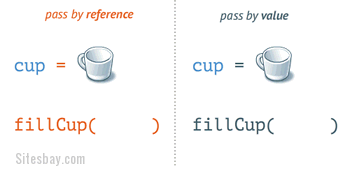# Function Arguments in C

## Call by Value and Call by Reference in C

On the basis of arguments there are two types of function are available in C language, they are;• With argument
• Without argument

If a function take any arguments, it must declare variables that accept the values as a arguments. These variables are called the formal parameters of the function. There are two ways to pass value or data to function in C language which is given below;

• call by value
• call by reference### GIF Animation

Now we can understand about call by reference and call by value by using Animated images.## Call by value

In call by value, original value can not be changed or modified. In call by value, when you passed value to the function it is locally stored by the function parameter in stack memory location. If you change the value of function parameter, it is changed for the current function only but it not change the value of variable inside the caller method such as main().

## Call by value

```
#include<stdio.h>
#include<conio.h>

void swap(int a, int b)
{
int temp;
temp=a;
a=b;
b=temp;
}

void main()
{
int a=100, b=200;
clrscr();
swap(a, b);  // passing value to function
printf("\nValue of a: %d",a);
printf("\nValue of b: %d",b);
getch();
}
```

```
Value of a: 200
Value of b: 100
```

## Call by reference

In call by reference, original value is changed or modified because we pass reference (address). Here, address of the value is passed in the function, so actual and formal arguments shares the same address space. Hence, any value changed inside the function, is reflected inside as well as outside the function.

## Example Call by reference

```
#include<stdio.h>
#include<conio.h>

void swap(int *a, int *b)
{
int temp;
temp=*a;
*a=*b;
*b=temp;
}

void main()
{
int a=100, b=200;
clrscr();
swap(&a, &b);  // passing value to function
printf("\nValue of a: %d",a);
printf("\nValue of b: %d",b);
getch();
}
```

## Output

```
Value of a: 200
Value of b: 100
```

### Difference between call by value and call by reference.

call by valuecall by reference
This method copy original value into function as a arguments.This method copy address of arguments into function as a arguments.
Changes made to the parameter inside the function have no effect on the argument.Changes made to the parameter affect the argument. Because address is used to access the actual argument.
Actual and formal arguments will be created in different memory locationActual and formal arguments will be created in same memory location

Note: By default, C uses call by value to pass arguments.

## Important points related to Function

• The basic purpose of the function is code reuse.
• From any function we can invoke (call) any another functions.
• Always compilation will be take place from top to bottom.
• Always execution process will starts from main() and ends with main() only.
• In implementation when we are calling a function which is define later for avoiding the compilation error we need to for forward declaration that is prototype is required.
• In function definition first line is called function declaration or function header.
• Always function declaration should be match with function declaratory.
• In implementation whenever a function does not returns any values back to the calling place then specify the return type.
• Void means nothing that is no return value.
• In implementation whenever a function returns other than void then specify the return type as return value type that is on e type of return value it is returning same type of return statement should be mentioned.
• Default return type of any function is an int.
• Default parameter type of any function is void.